# Rajasthan Board Question Paper for Class 10 Maths 2019 In PDF

## RBSE 10th Maths Question Papers 2019 With Solutions

Rajasthan Board (RBSE) Class 10 Maths 2019 question paper with solutions are provided here in a downloadable pdf format and also in the text format so that the students can easily access them. Along with the solutions, they can also get the Rajasthan Board Class 10 Maths Question Paper 2019 for reference. Students are able to access all the Rajasthan board previous year maths question papers here. RBSE Class 10th Maths question paper 2019 can be downloaded easily, and students can practice and verify the answers provided by BYJU’S. Solving 2019 Maths question papers for class 10 RBSE Board will help the students to predict what type of questions will appear in the exam.

### QUESTION PAPER CODE S–09–Mathematics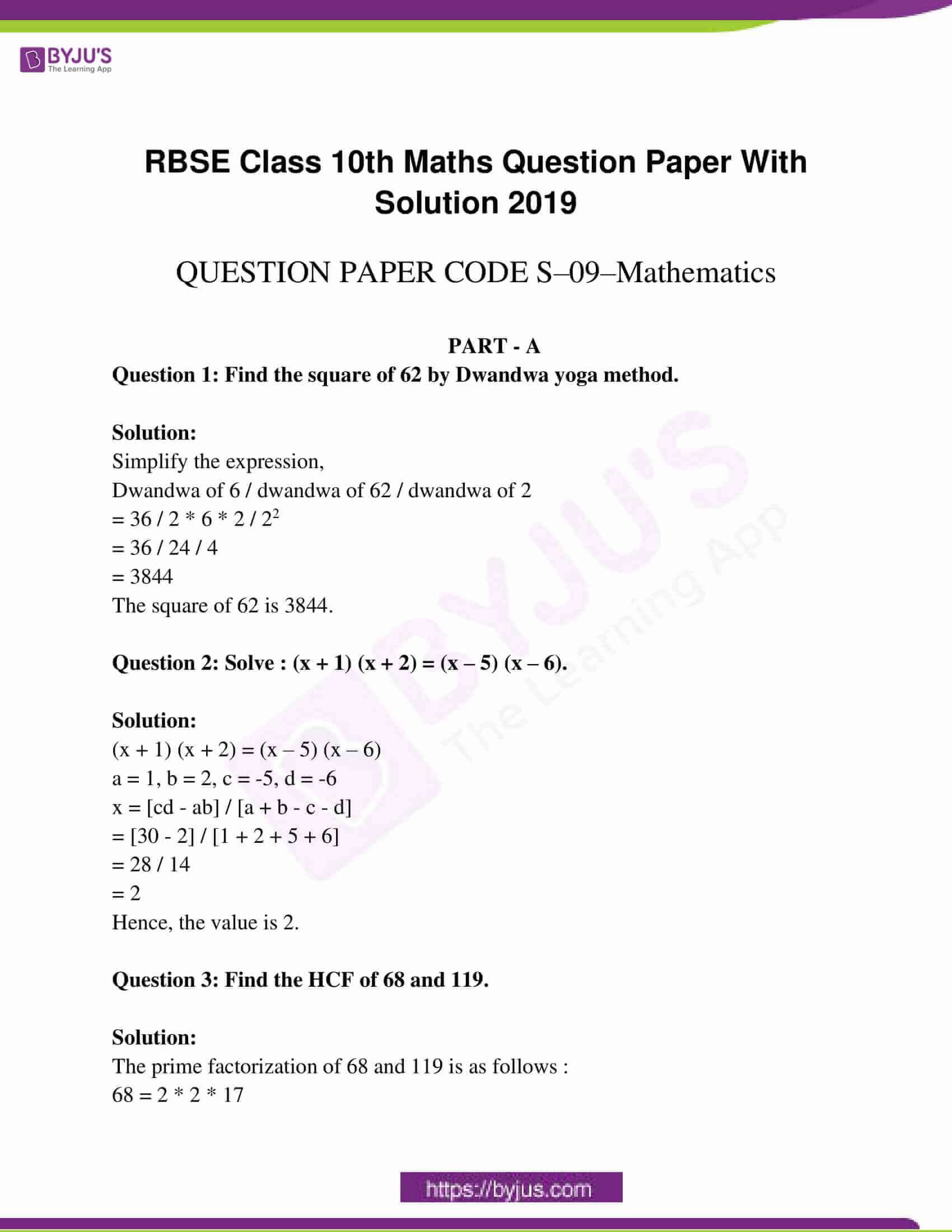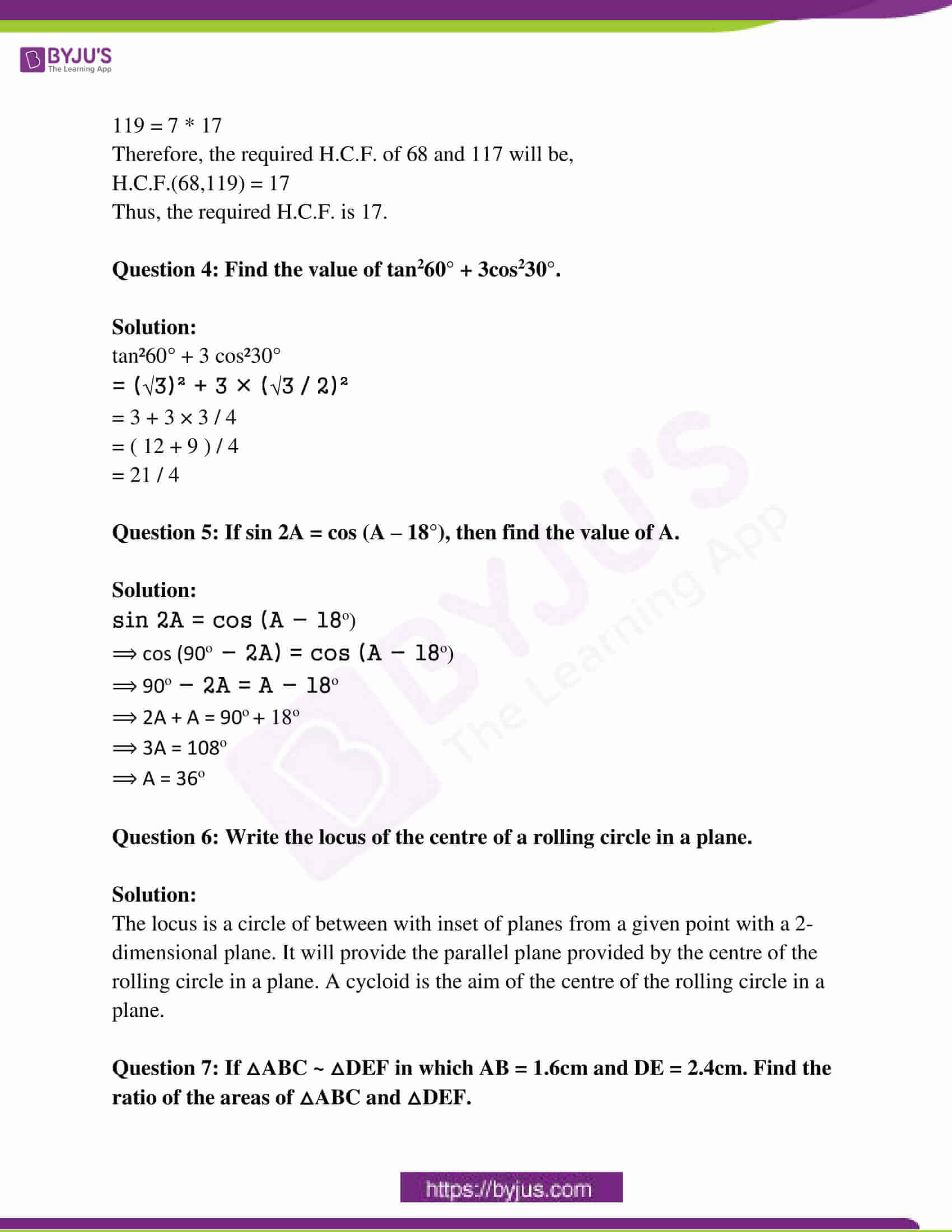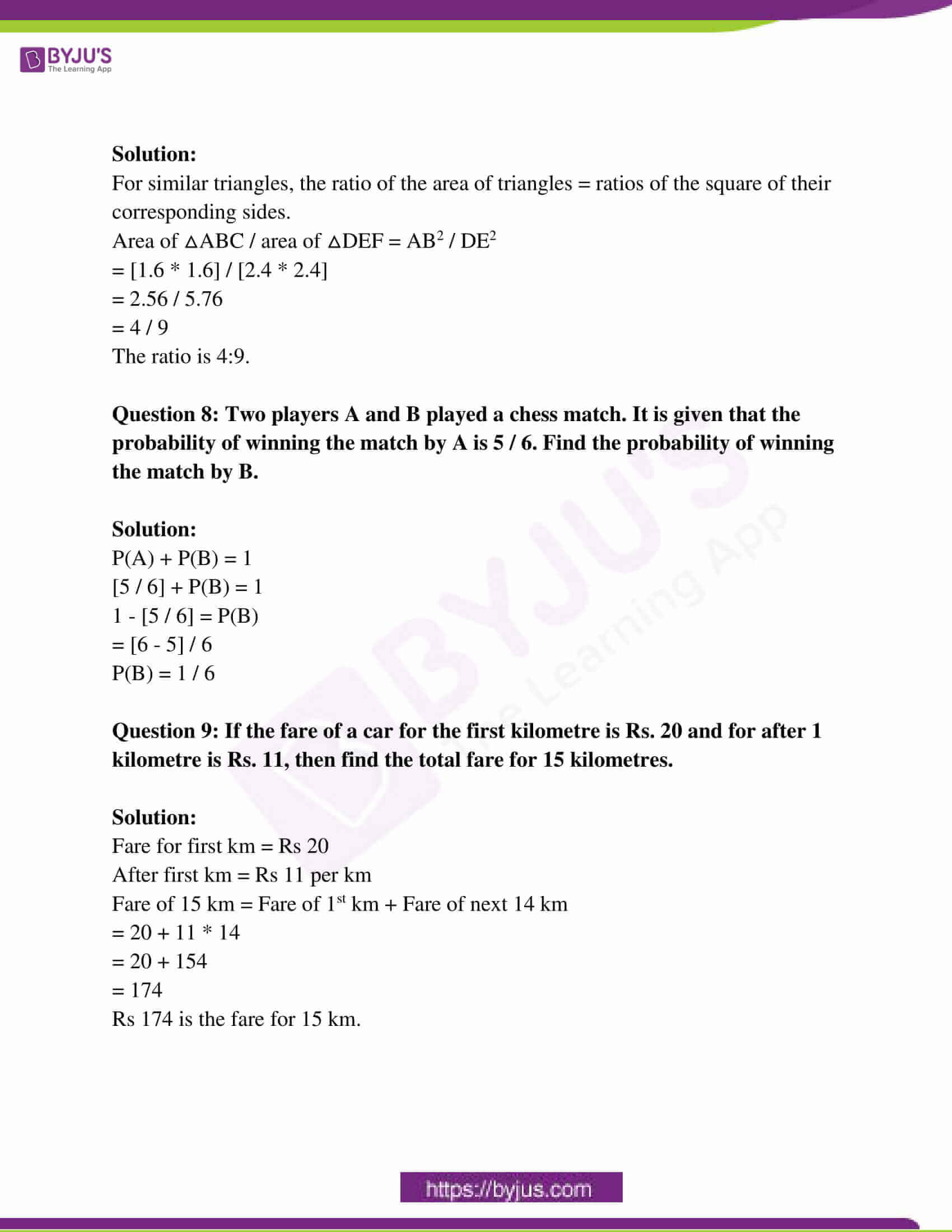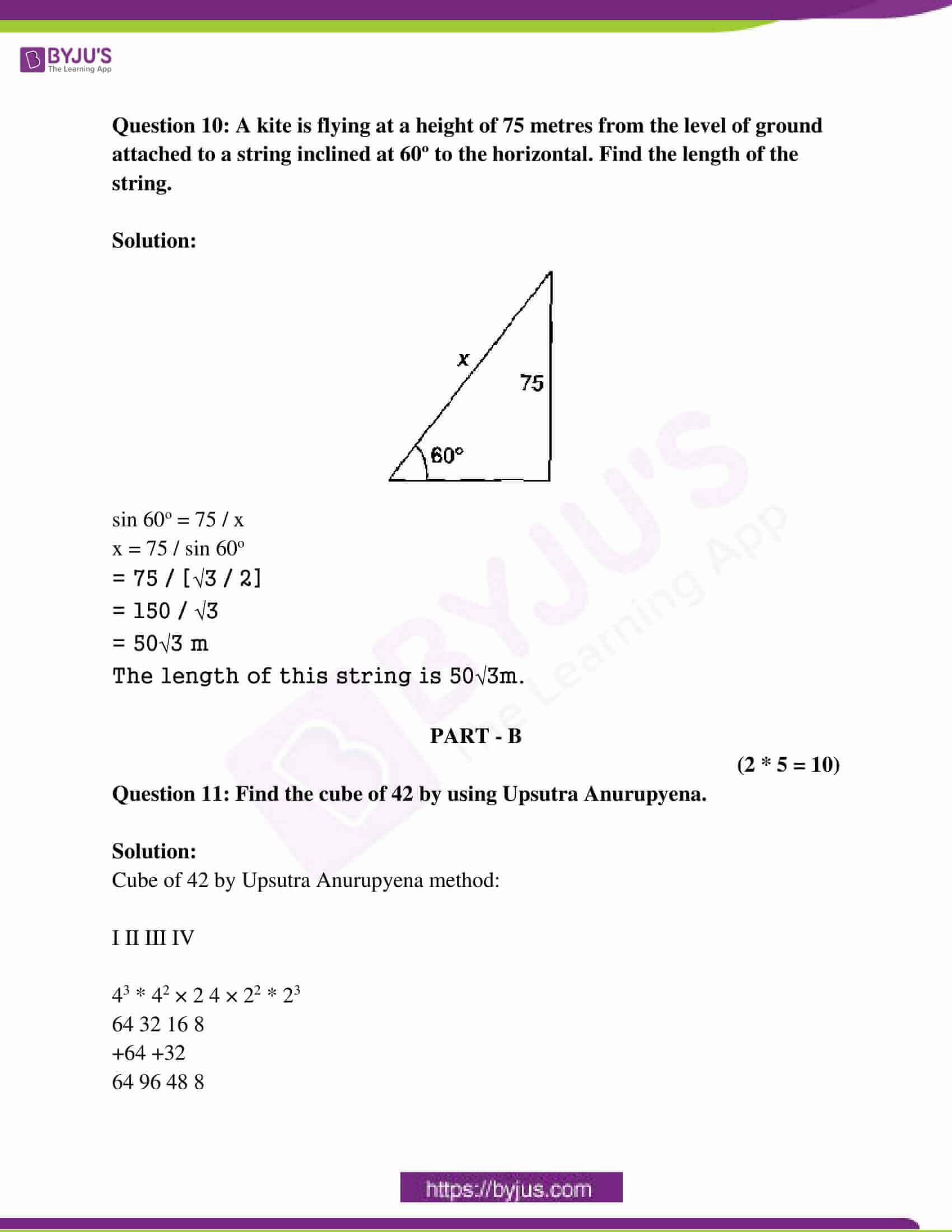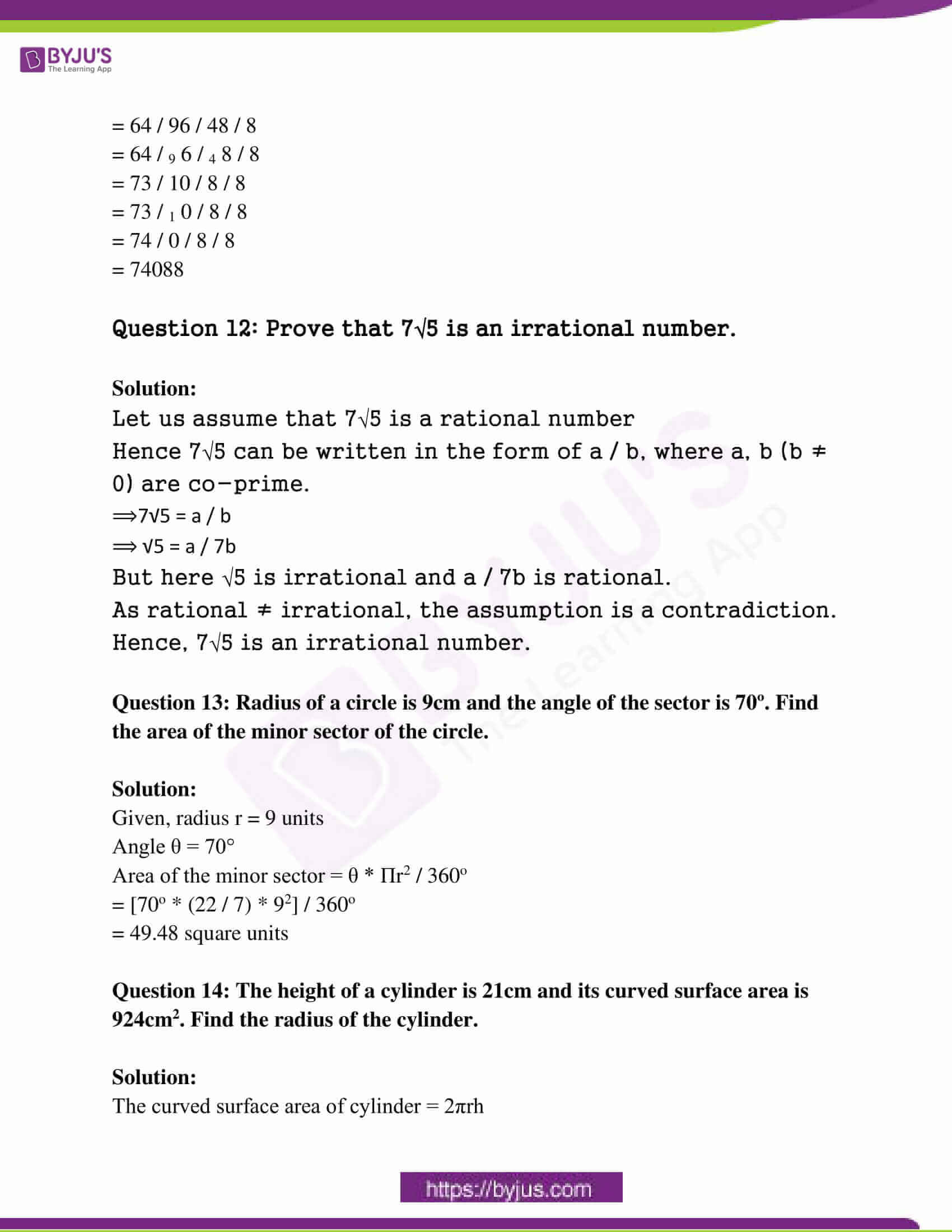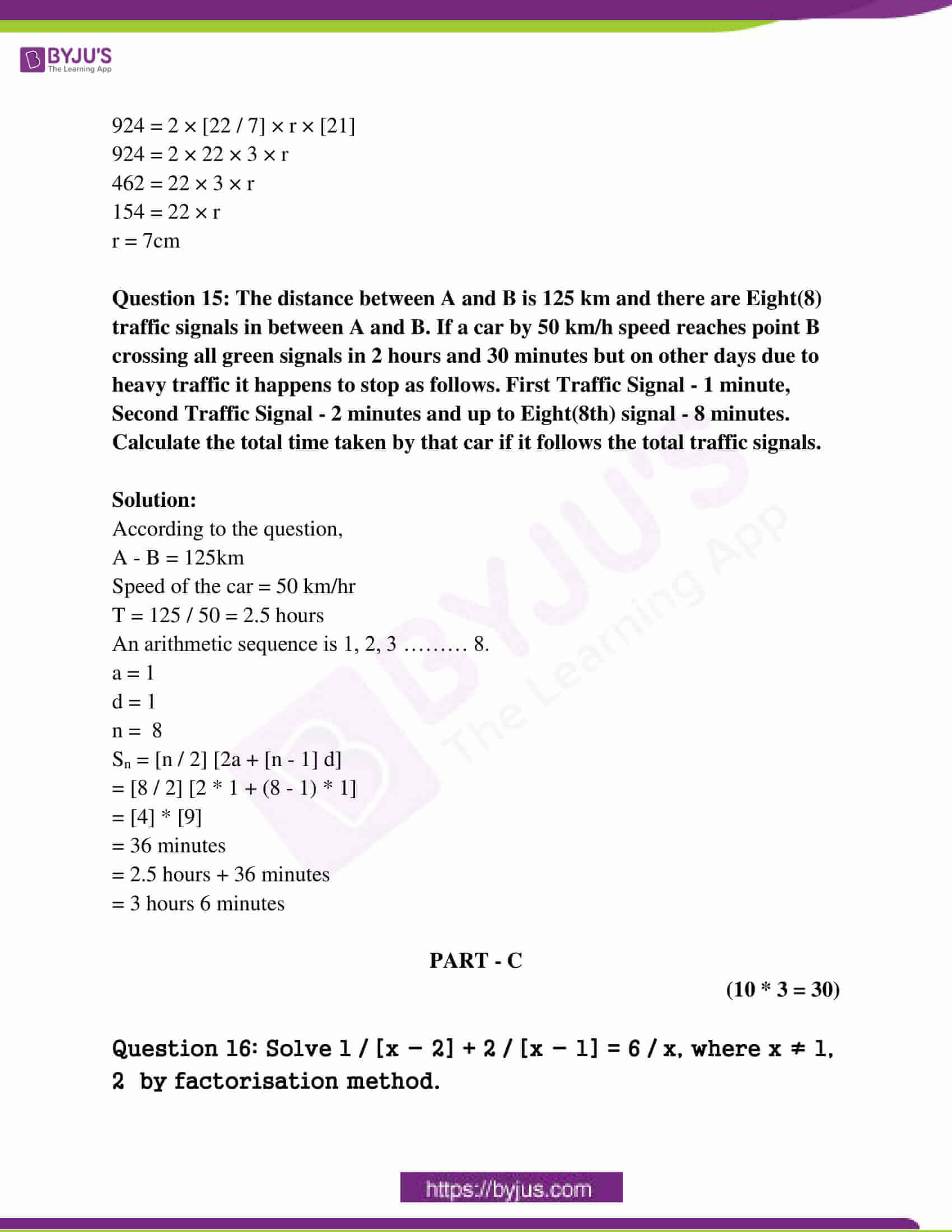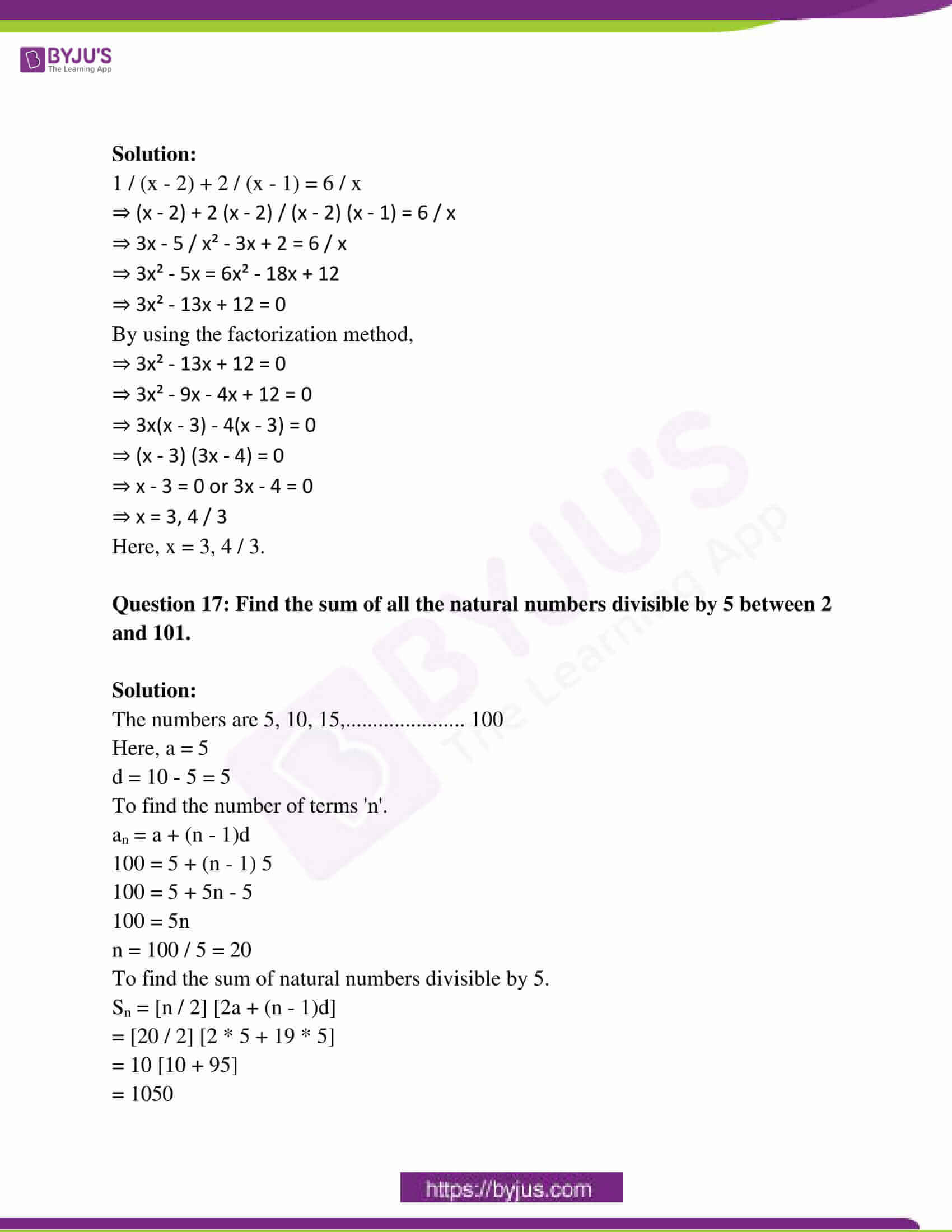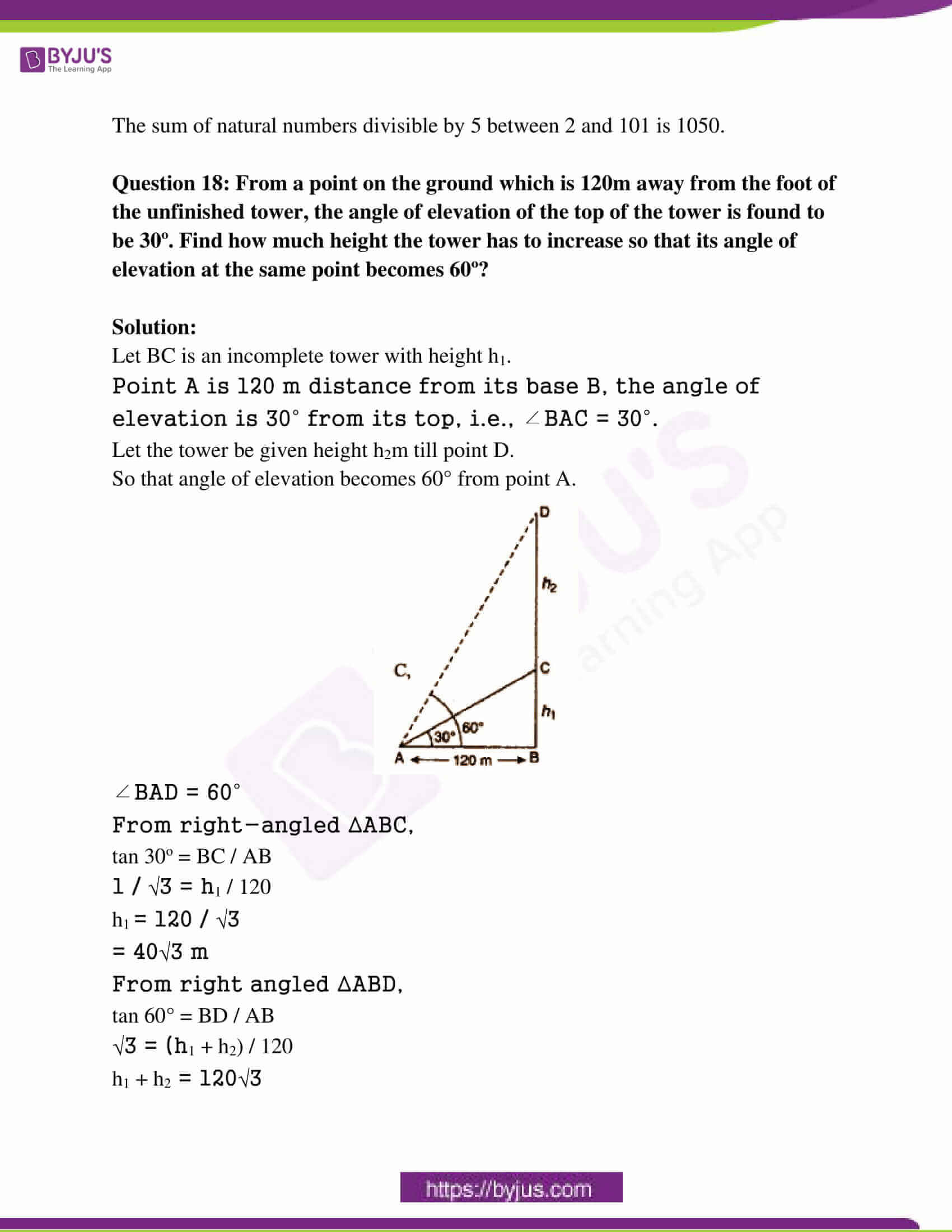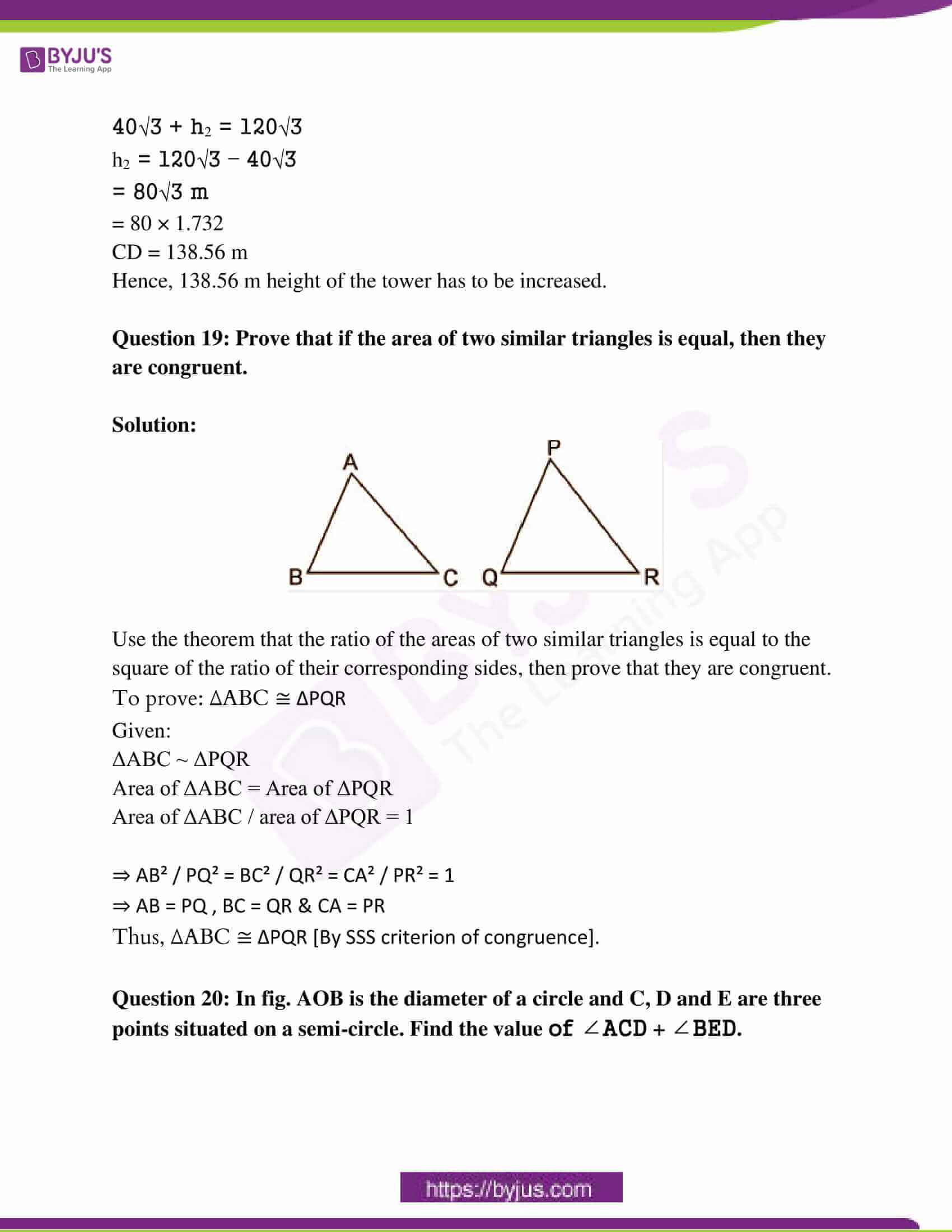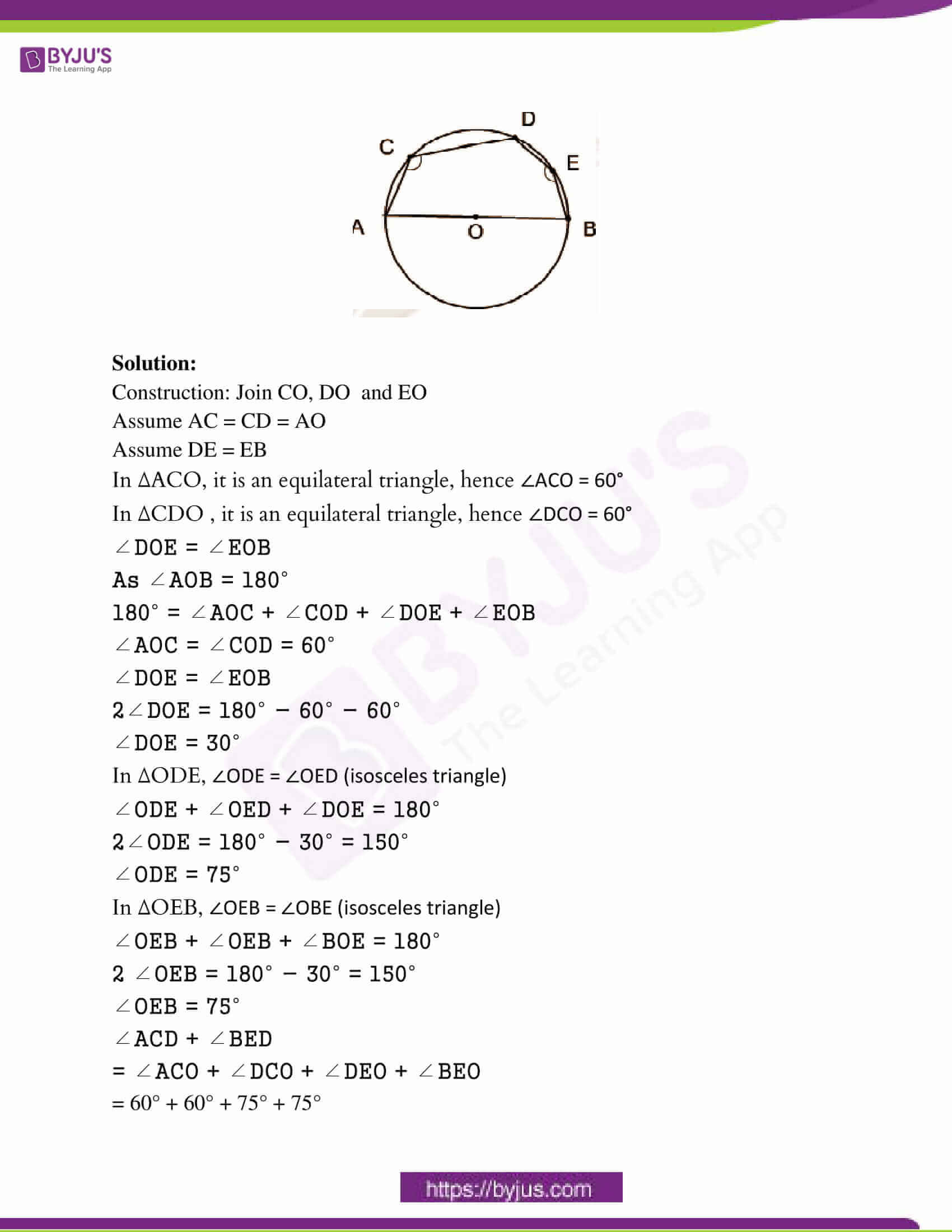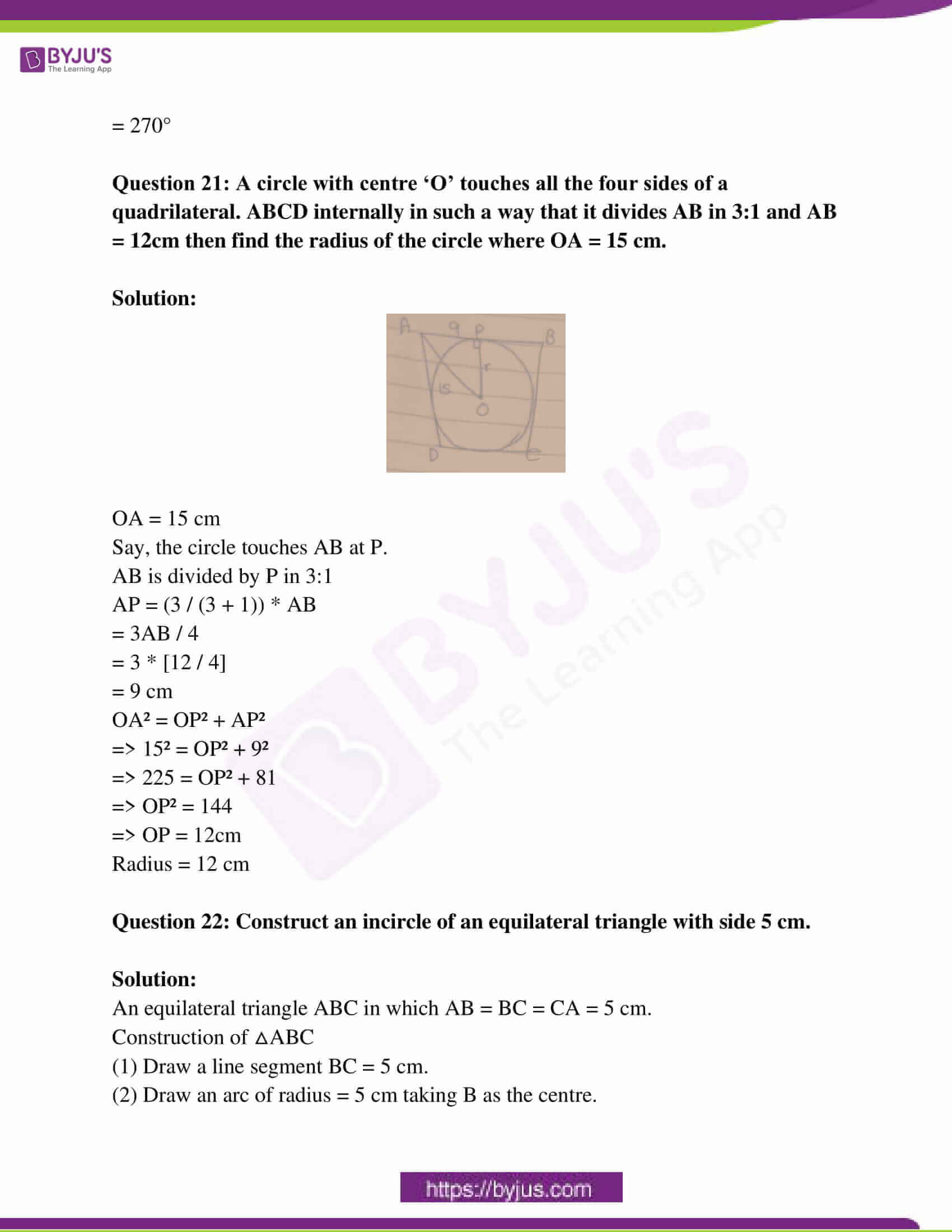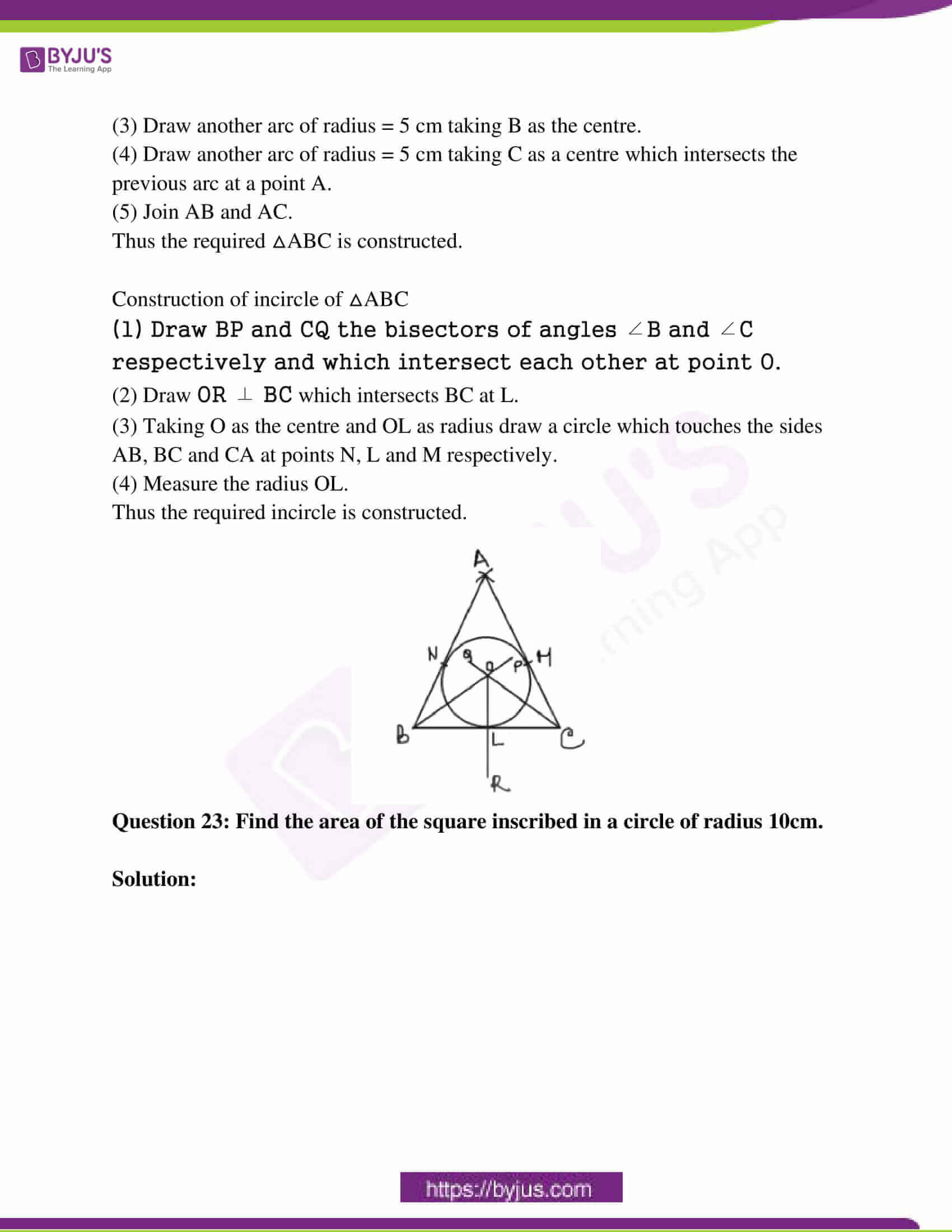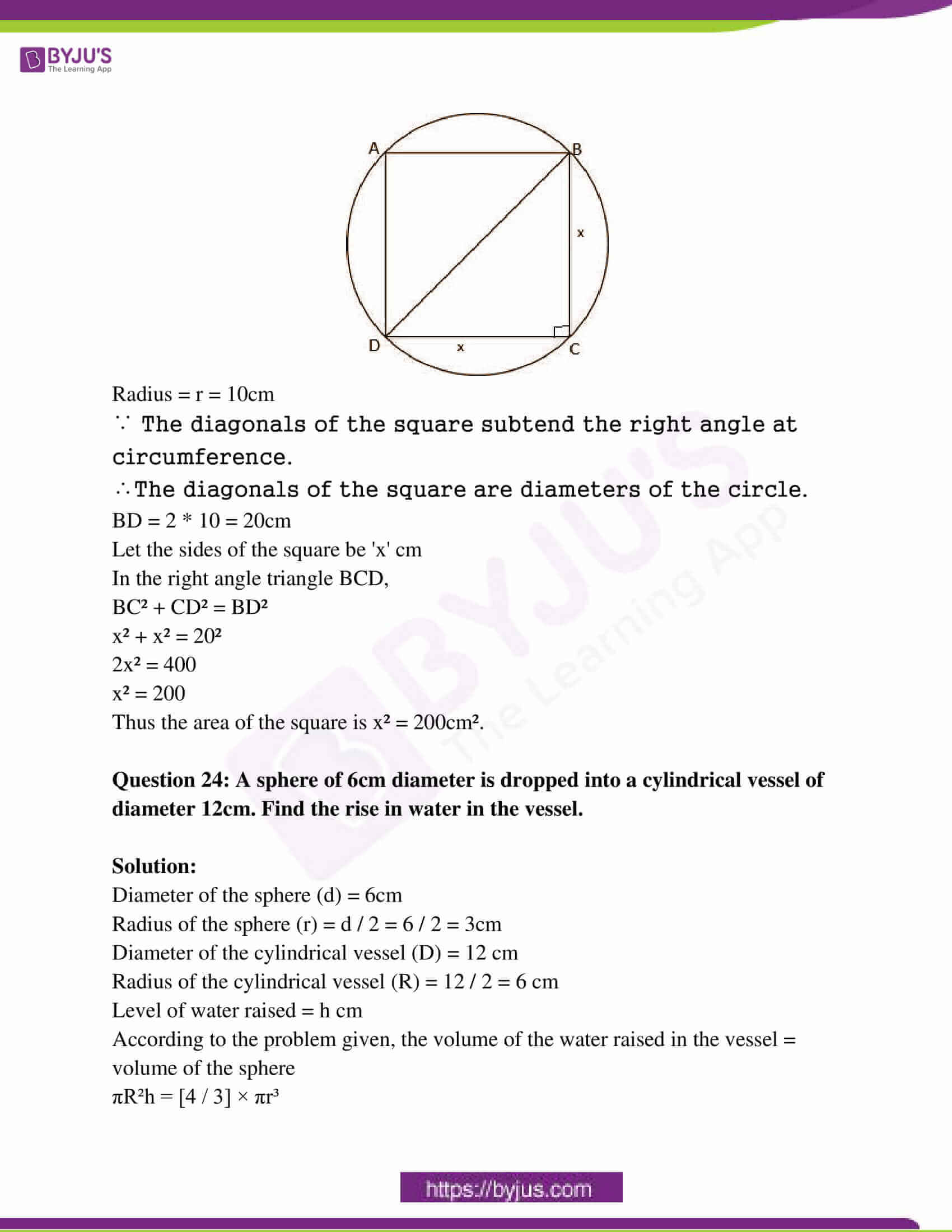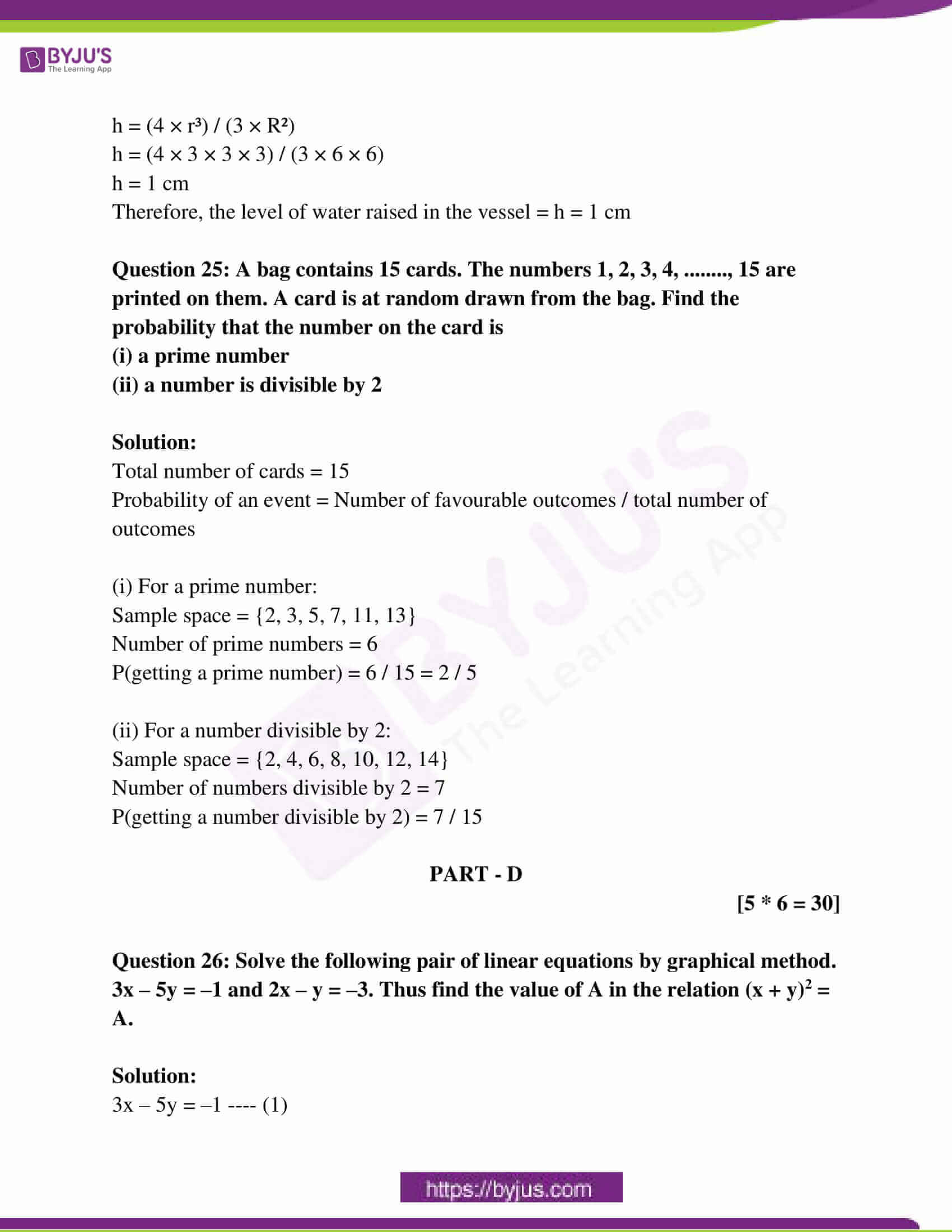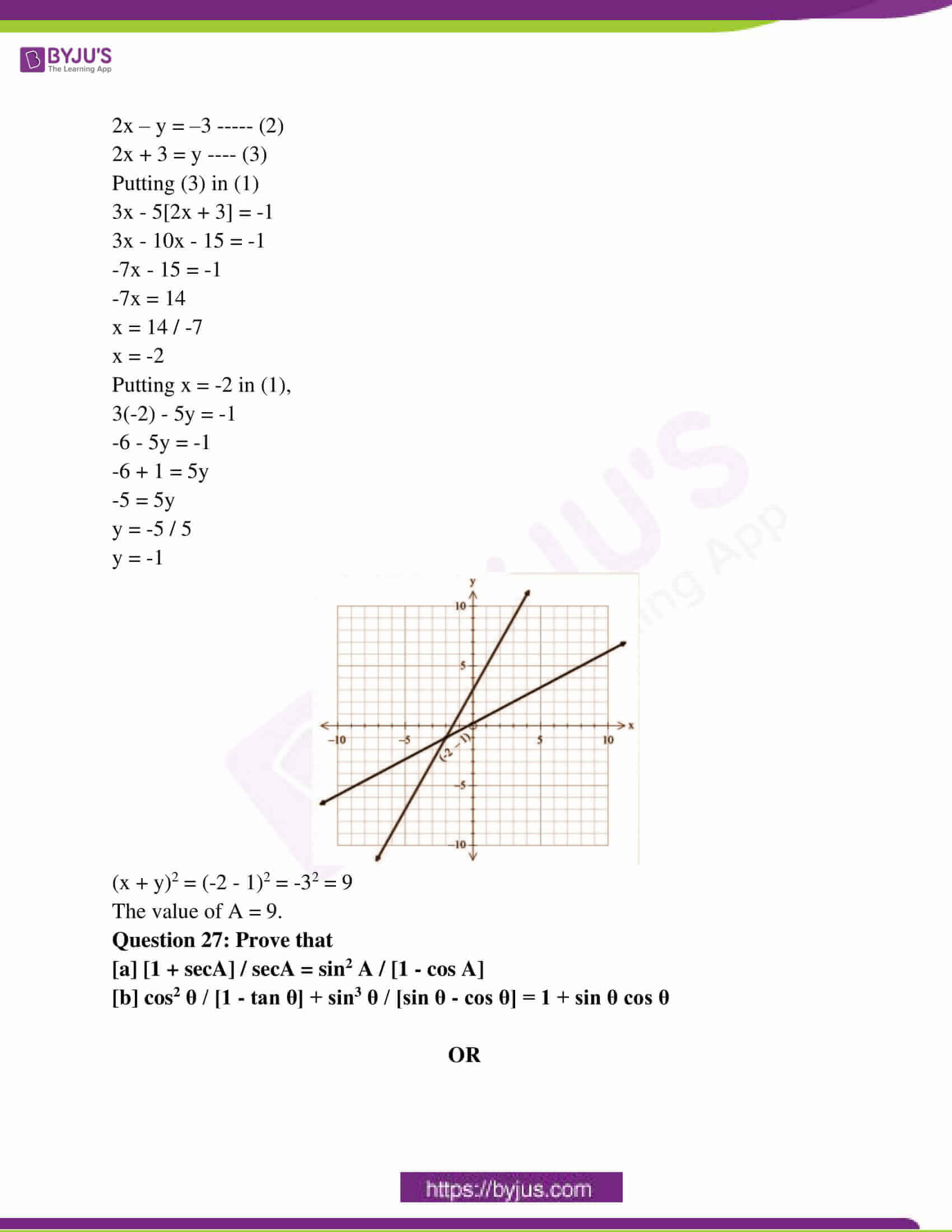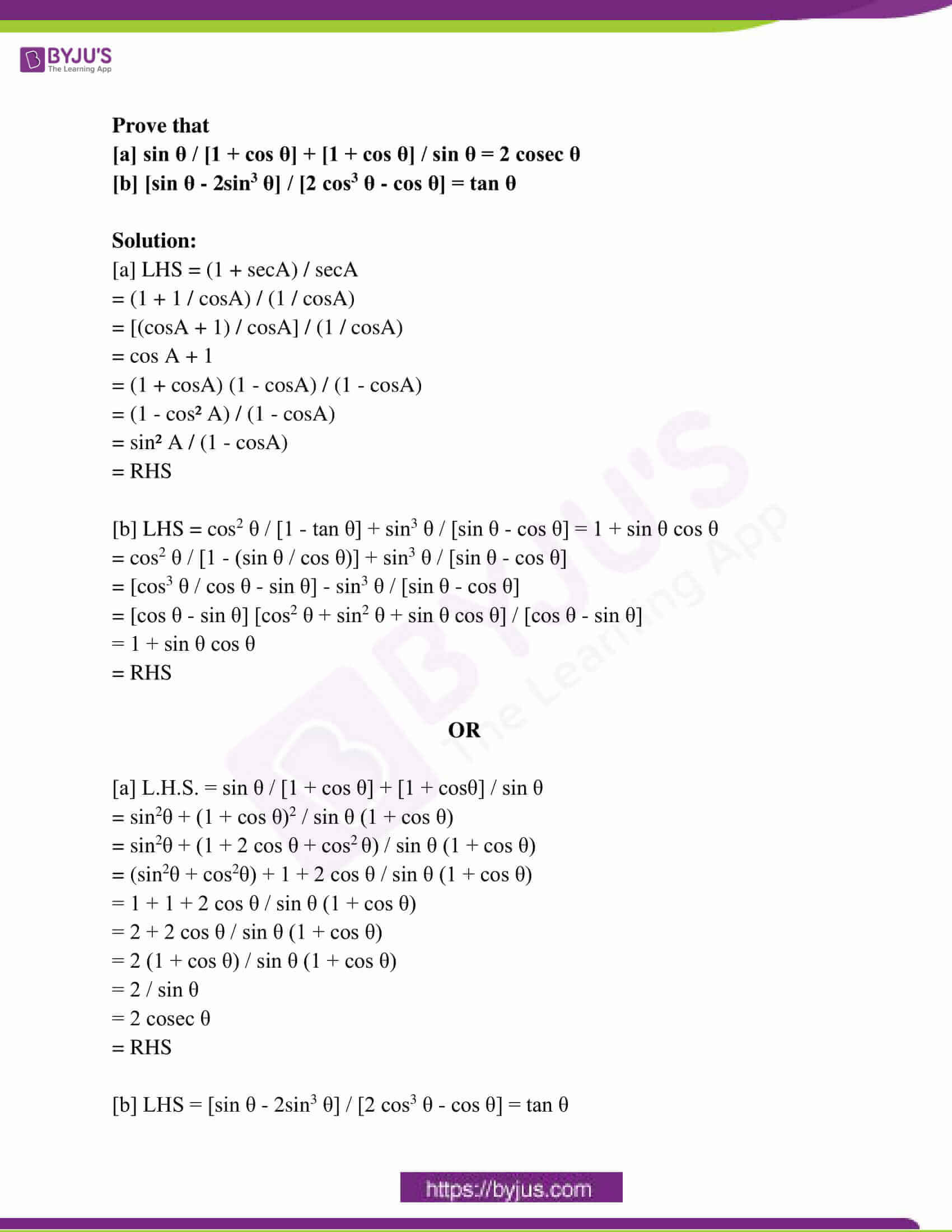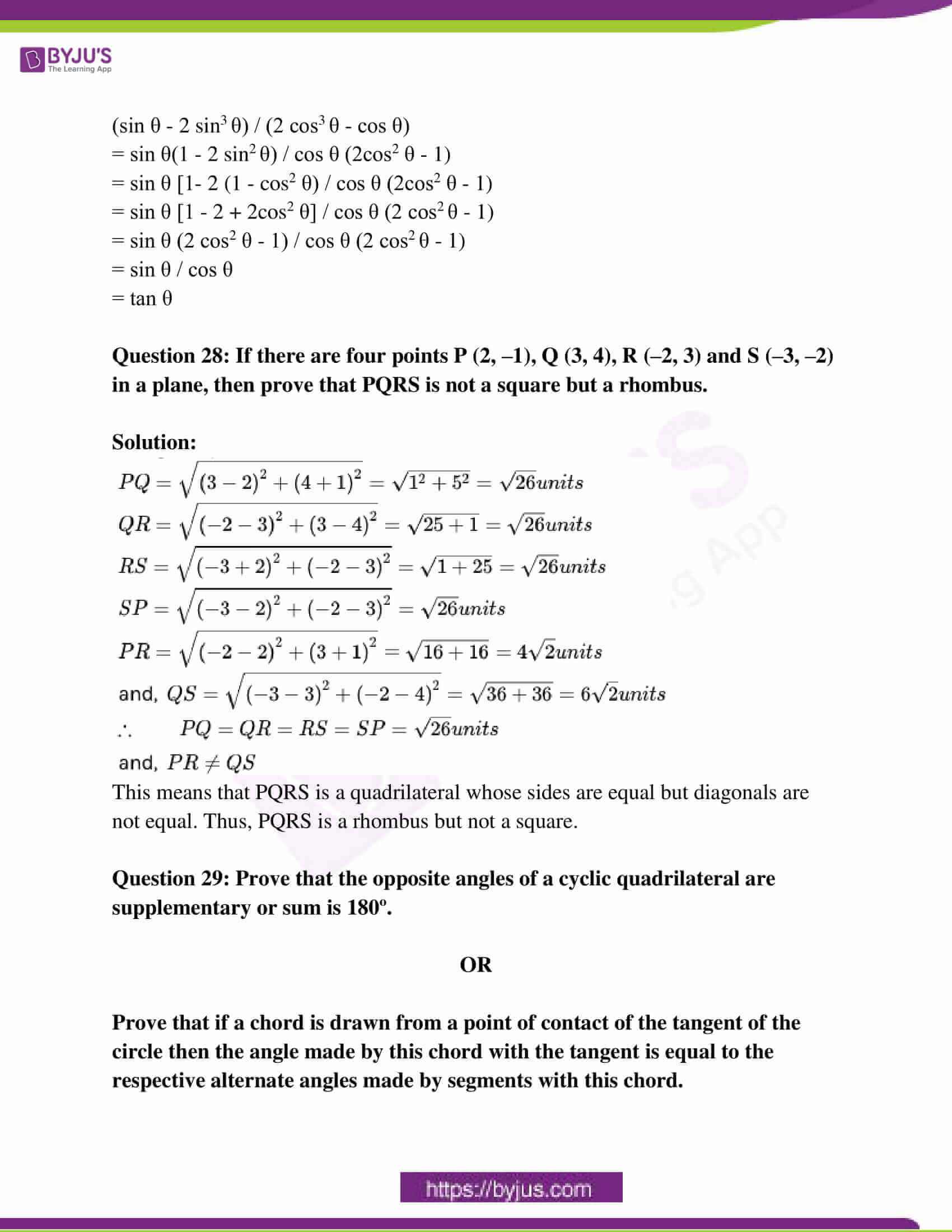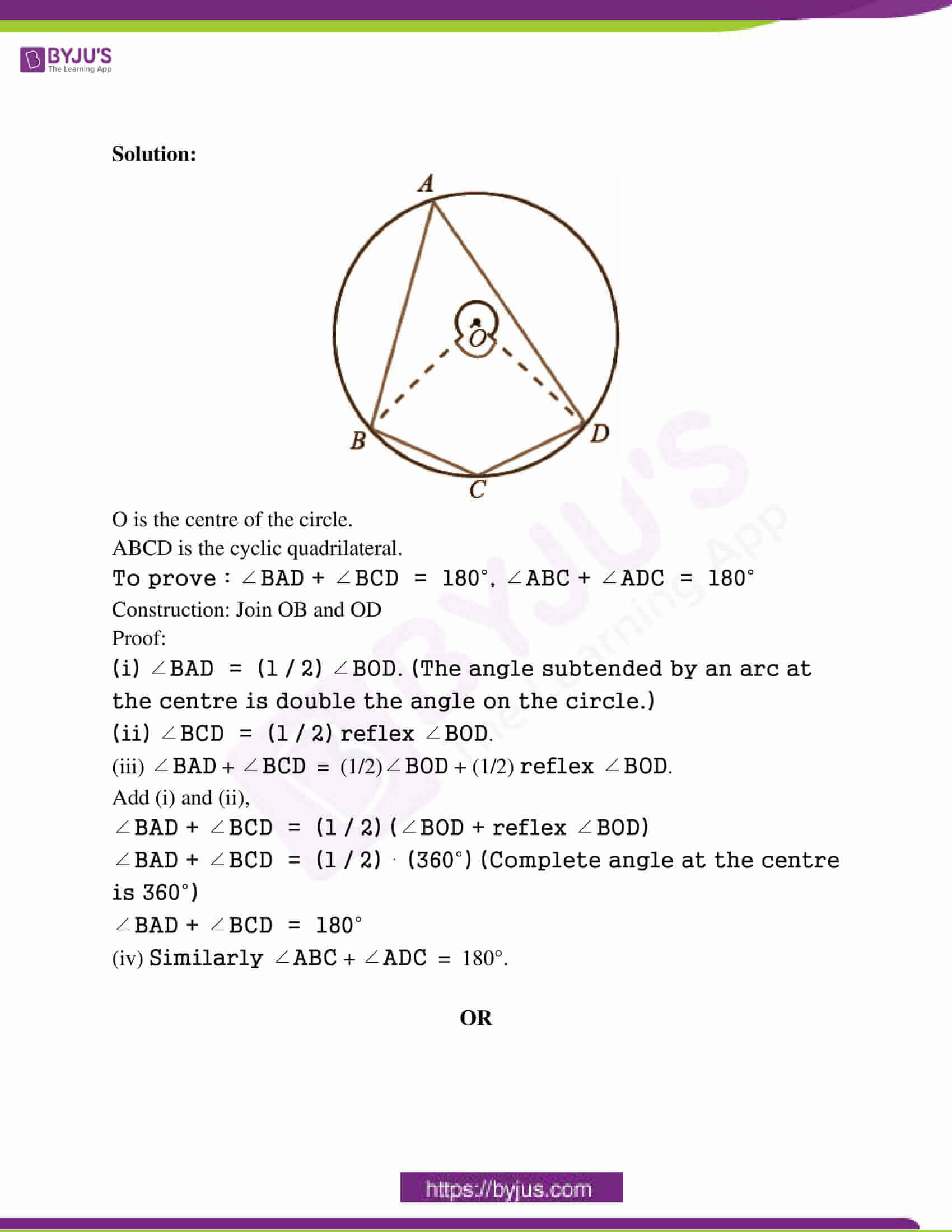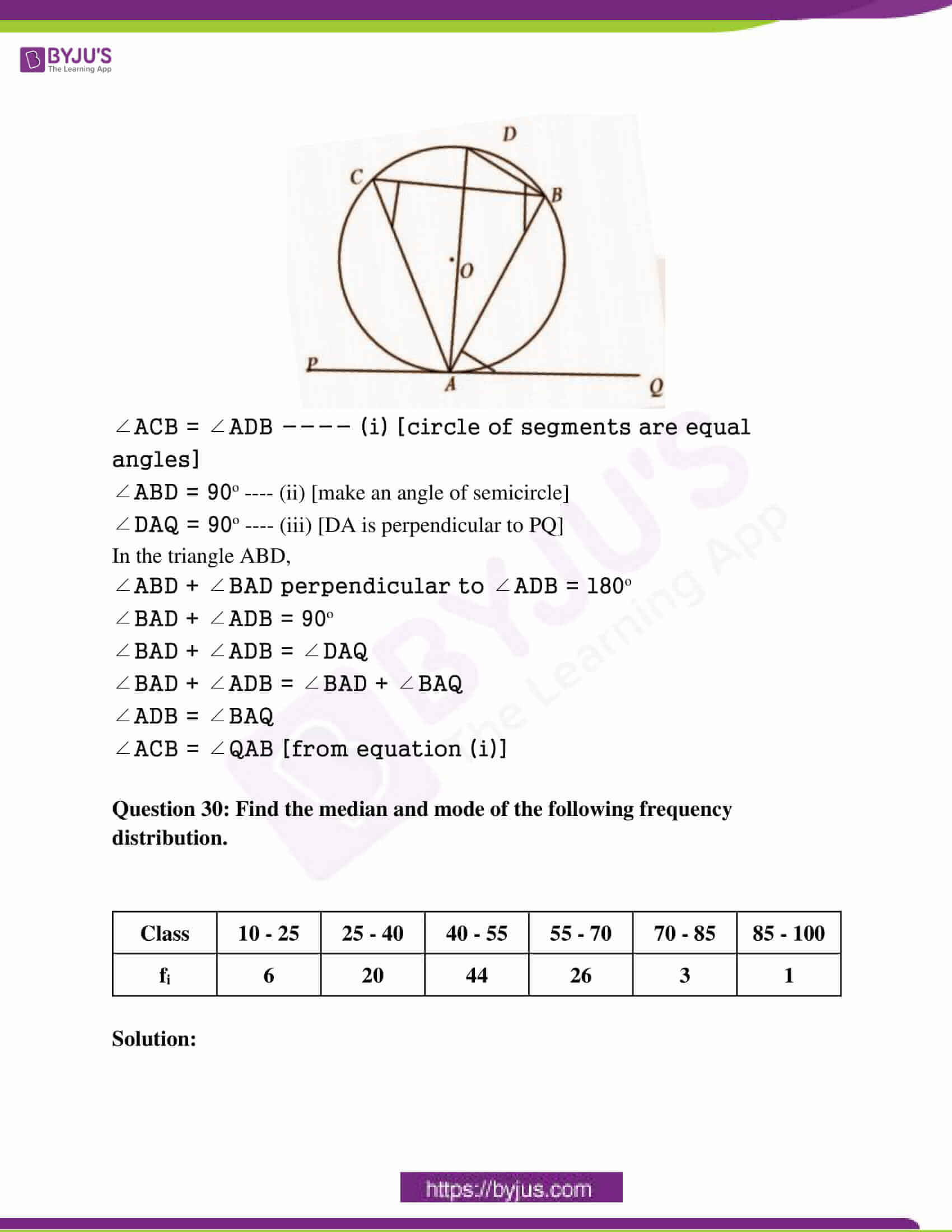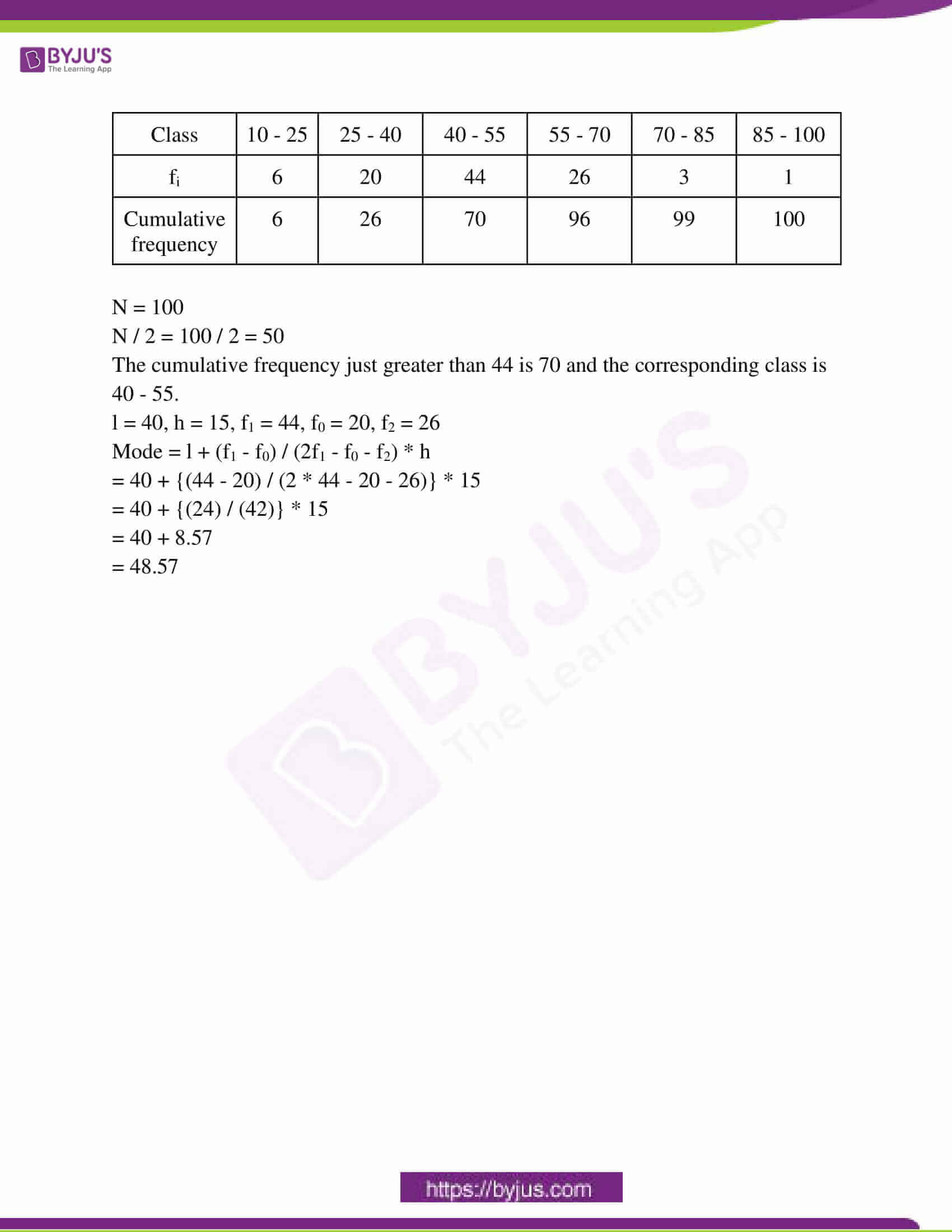### RBSE Class 10th Maths Question Paper With Solution 2019

PART – A

Question 1: Find the square of 62 by Dwandwa yoga method.

Solution:

Simplify the expression,

Dwandwa of 6 / dwandwa of 62 / dwandwa of 2

= 36 / 2 * 6 * 2 / 22

= 36 / 24 / 4

= 3844

The square of 62 is 3844.

Question 2: Solve : (x + 1) (x + 2) = (x – 5) (x – 6).

Solution:

(x + 1) (x + 2) = (x – 5) (x – 6)

a = 1, b = 2, c = -5, d = -6

x = [cd – ab] / [a + b – c – d]

= [30 – 2] / [1 + 2 + 5 + 6]

= 28 / 14

= 2

Hence, the value is 2.

Question 3: Find the HCF of 68 and 119.

Solution:

The prime factorization of 68 and 119 is as follows :

68 = 2 * 2 * 17

119 = 7 * 17

Therefore, the required H.C.F. of 68 and 117 will be,

H.C.F.(68,119) = 17

Thus, the required H.C.F. is 17.

Question 4: Find the value of tan260° + 3cos230°.

Solution:

tan²60° + 3 cos²30°

= (√3)² + 3 × (√3 / 2)²

= 3 + 3 × 3 / 4

= ( 12 + 9 ) / 4

= 21 / 4

Question 5: If sin 2A = cos (A – 18°), then find the value of A.

Solution:

sin 2A = cos (A − 18o)

⟹ cos (90o − 2A) = cos (A − 18o)

⟹ 90o − 2A = A − 18o

⟹ 2A + A = 90o + 18o

⟹ 3A = 108o

⟹ A = 36o

Question 6: Write the locus of the centre of a rolling circle in a plane.

Solution:

The locus is a circle of between with inset of planes from a given point with a 2- dimensional plane. It will provide the parallel plane provided by the centre of the rolling circle in a plane. A cycloid is the aim of the centre of the rolling circle in a plane.

Question 7: If △ABC ~ △DEF in which AB = 1.6cm and DE = 2.4cm. Find the ratio of the areas of △ABC and △DEF.

Solution:

For similar triangles, the ratio of the area of triangles = ratios of the square of their corresponding sides.

Area of △ABC / area of △DEF = AB2 / DE2

= [1.6 * 1.6] / [2.4 * 2.4]

= 2.56 / 5.76

= 4 / 9

The ratio is 4:9.

Question 8: Two players A and B played a chess match. It is given that the probability of winning the match by A is 5 / 6. Find the probability of winning the match by B.

Solution:

P(A) + P(B) = 1

[5 / 6] + P(B) = 1

1 – [5 / 6] = P(B)

= [6 – 5] / 6

P(B) = 1 / 6

Question 9: If the fare of a car for the first kilometre is Rs. 20 and for after 1 kilometre it is Rs. 11, then find the total fare for 15 kilometres.

Solution:

Fare for first km = Rs 20

After first km = Rs 11 per km

Fare of 15 km = Fare of 1st km + Fare of next 14 km

= 20 + 11 * 14

= 20 + 154

= 174

Rs 174 is the fare for 15 km.

Question 10: A kite is flying at a height of 75 metres from the level of ground attached to a string inclined at 60º to the horizontal. Find the length of the string.

Solution: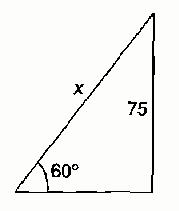sin 60o = 75 / x

x = 75 / sin 60o

= 75 / [√3 / 2]

= 150 / √3

= 50√3 m

The length of this string is 50√3m.

PART – B

(2 * 5 = 10)

Question 11: Find the cube of 42 by using Upsutra Anurupyena.

Solution:

Cube of 42 by Upsutra Anurupyena method:

I II III IV

43 * 42 × 2 4 × 22 * 23

64 32 16 8

+64 +32

64 96 48 8

= 64 / 96 / 48 / 8

= 64 / 9 6 / 4 8 / 8

= 73 / 10 / 8 / 8

= 73 / 1 0 / 8 / 8

= 74 / 0 / 8 / 8

= 74088

Question 12: Prove that 7√5 is an irrational number.

Solution:

Let us assume that 7√5 is a rational number

Hence 7√5 can be written in the form of a / b, where a, b (b ≠ 0) are co-prime.

⟹7√5 = a / b

⟹ √5 = a / 7b

But here √5 is irrational and a / 7b is rational.

As rational ≠ irrational, the assumption is a contradiction.

Hence, 7√5 is an irrational number.

Question 13: Radius of a circle is 9cm and the angle of the sector is 70º. Find the area of the minor sector of the circle.

Solution:

Given, radius r = 9 units

Angle θ = 70°

Area of the minor sector = θ * Πr2 / 360o

= [70o * (22 / 7) * 92] / 360o

= 49.48 square units

Question 14: The height of a cylinder is 21cm and its curved surface area is 924cm2. Find the radius of the cylinder.

Solution:

The curved surface area of cylinder = 2πrh

924 = 2 × [22 / 7] × r × 

924 = 2 × 22 × 3 × r

462 = 22 × 3 × r

154 = 22 × r

r = 7cm

Question 15: The distance between A and B is 125 km and there are Eight (8) traffic signals in between A and B. If a car by 50 km/h speed reaches point B crossing all green signals in 2 hours and 30 minutes but on other days due to heavy traffic it happens to stop as follows. First Traffic Signal – 1 minute, Second Traffic Signal – 2 minutes and up to Eight (8th) signal – 8 minutes. Calculate the total time taken by that car if it follows the total traffic signals.

Solution:

According to the question,

A – B = 125km

Speed of the car = 50 km/hr

T = 125 / 50 = 2.5 hours

An arithmetic sequence is 1, 2, 3 ……… 8.

a = 1

d = 1

n = 8

Sn = [n / 2] [2a + [n – 1] d]

= [8 / 2] [2 * 1 + (8 – 1) * 1]

=  * 

= 36 minutes

= 2.5 hours + 36 minutes

= 3 hours 6 minutes

PART – C

(10 * 3 = 30)

Question 16: Solve 1 / [x – 2] + 2 / [x – 1] = 6 / x, where x ≠ 1, 2 by factorisation method.

Solution:

1 / (x – 2) + 2 / (x – 1) = 6 / x

⇒ (x – 2) + 2 (x – 2) / (x – 2) (x – 1) = 6 / x

⇒ 3x – 5 / x² – 3x + 2 = 6 / x

⇒ 3x² – 5x = 6x² – 18x + 12

⇒ 3x² – 13x + 12 = 0

By using the factorization method,

⇒ 3x² – 13x + 12 = 0

⇒ 3x² – 9x – 4x + 12 = 0

⇒ 3x(x – 3) – 4(x – 3) = 0

⇒ (x – 3) (3x – 4) = 0

⇒ x – 3 = 0 or 3x – 4 = 0

⇒ x = 3, 4 / 3

Here, x = 3, 4 / 3.

Question 17: Find the sum of all the natural numbers divisible by 5 between 2 and 101.

Solution:

The numbers are 5, 10, 15,…………………. 100

Here, a = 5

d = 10 – 5 = 5

To find the number of terms ‘n’.

an = a + (n – 1)d

100 = 5 + (n – 1) 5

100 = 5 + 5n – 5

100 = 5n

n = 100 / 5 = 20

To find the sum of natural numbers divisible by 5.

Sn = [n / 2] [2a + (n – 1)d]

= [20 / 2] [2 * 5 + 19 * 5]

= 10 [10 + 95]

= 1050

The sum of natural numbers divisible by 5 between 2 and 101 is 1050.

Question 18: From a point on the ground which is 120m away from the foot of the unfinished tower, the angle of elevation of the top of the tower is found to be 30º. Find how much height the tower has to increase so that its angle of elevation at the same point becomes 60º?

Solution:

Let BC is an incomplete tower with height h1.

Point A is 120 m distance from its base B, the angle of elevation is 30° from its top, i.e., ∠BAC = 30°.

Let the tower be given height h2m till point D.

So that angle of elevation becomes 60° from point A.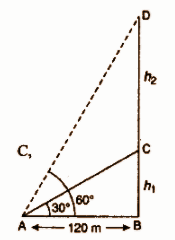From right-angled ∆ABC,

tan 30o = BC / AB

1 / √3 = h1 / 120

h1 = 120 / √3

= 40√3 m

From right angled ∆ABD,

tan 60° = BD / AB

√3 = (h1 + h2) / 120

h1 + h2 = 120√3

40√3 + h2 = 120√3

h2 = 120√3 – 40√3

= 80√3 m

= 80 × 1.732

CD = 138.56 m

Hence, 138.56 m height of the tower has to be increased.

Question 19: Prove that if the area of two similar triangles is equal, then they are congruent.

Solution: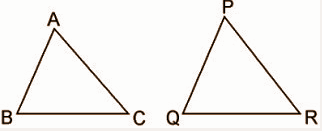Use the theorem that the ratio of the areas of two similar triangles is equal to the square of the ratio of their corresponding sides, then prove that they are congruent.

To prove: ΔABC ≅ ΔPQR

Given:

ΔABC ~ ΔPQR

Area of ΔABC = Area of ΔPQR

Area of ΔABC / area of ΔPQR = 1

⇒ AB² / PQ² = BC² / QR² = CA² / PR² = 1

⇒ AB = PQ , BC = QR & CA = PR

Thus, ΔABC ≅ ΔPQR [By SSS criterion of congruence].

Question 20: In fig. AOB is the diameter of a circle and C, D and E are three points situated on a semi-circle. Find the value of ∠ACD + ∠BED.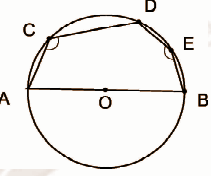Solution:

Construction: Join CO, DO and EO

Assume AC = CD = AO

Assume DE = EB

In ΔACO, it is an equilateral triangle, hence ∠ACO = 60°

In ΔCDO , it is an equilateral triangle, hence ∠DCO = 60°

∠DOE = ∠EOB

As ∠AOB = 180°

180° = ∠AOC + ∠COD + ∠DOE + ∠EOB

∠AOC = ∠COD = 60°

∠DOE = ∠EOB

2∠DOE = 180° – 60° – 60°

∠DOE = 30°

In ΔODE, ∠ODE = ∠OED (isosceles triangle)

∠ODE + ∠OED + ∠DOE = 180°

2∠ODE = 180° – 30° = 150°

∠ODE = 75°

In ΔOEB, ∠OEB = ∠OBE (isosceles triangle)

∠OEB + ∠OEB + ∠BOE = 180°

2 ∠OEB = 180° – 30° = 150°

∠OEB = 75°

∠ACD + ∠BED

= ∠ACO + ∠DCO + ∠DEO + ∠BEO

= 60° + 60° + 75° + 75°

= 270°

Question 21: A circle with centre ‘O’ touches all the four sides of a quadrilateral. ABCD internally in such a way that it divides AB in 3:1 and AB = 12cm then find the radius of the circle where OA = 15 cm.

Solution: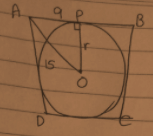OA = 15 cm

Say, the circle touches AB at P.

AB is divided by P in 3:1

AP = (3 / (3 + 1)) * AB

= 3AB / 4

= 3 * [12 / 4]

= 9 cm

OA² = OP² + AP²

=> 15² = OP² + 9²

=> 225 = OP² + 81

=> OP² = 144

=> OP = 12cm

Question 22: Construct an incircle of an equilateral triangle with side 5 cm.

Solution:

An equilateral triangle ABC in which AB = BC = CA = 5 cm.

Construction of △ABC

(1) Draw a line segment BC = 5 cm.

(2) Draw an arc of radius = 5 cm taking B as the centre.

(3) Draw another arc of radius = 5 cm taking B as the centre.

(4) Draw another arc of radius = 5 cm taking C as a centre which intersects the previous arc at a point A.

(5) Join AB and AC.

Thus the required △ABC is constructed.

Construction of incircle of △ABC

(1) Draw BP and CQ the bisectors of angles ∠B and ∠C respectively and which intersect each other at point O.

(2) Draw OR ⊥ BC which intersects BC at L.

(3) Taking O as the centre and OL as radius draw a circle which touches the sides AB, BC and CA at points N, L and M respectively.

Thus the required incircle is constructed.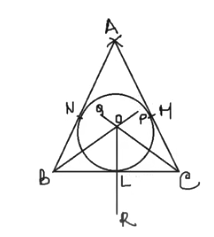Question 23: Find the area of the square inscribed in a circle of radius 10cm.

Solution: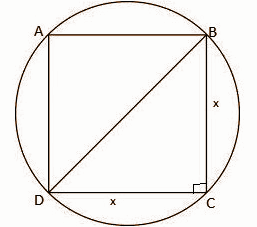∵ The diagonals of the square subtend the right angle at circumference.

∴The diagonals of the square are diameters of the circle.

BD = 2 * 10 = 20cm

Let the sides of the square be ‘x’ cm

In the right angle triangle BCD,

BC² + CD² = BD²

x² + x² = 20²

2x² = 400

x² = 200

Thus the area of the square is x² = 200cm².

Question 24: A sphere of 6cm diameter is dropped into a cylindrical vessel of diameter 12cm. Find the rise in water in the vessel.

Solution:

Diameter of the sphere (d) = 6cm

Radius of the sphere (r) = d / 2 = 6 / 2 = 3cm

Diameter of the cylindrical vessel (D) = 12 cm

Radius of the cylindrical vessel (R) = 12 / 2 = 6 cm

Level of water raised = h cm

According to the problem given, the volume of the water raised in the vessel = volume of the sphere

πR²h = [4 / 3] × πr³

h = (4 × r³) / (3 × R²)

h = (4 × 3 × 3 × 3) / (3 × 6 × 6)

h = 1 cm

Therefore, the level of water raised in the vessel = h = 1 cm

Question 25: A bag contains 15 cards. The numbers 1, 2, 3, 4, …….., 15 are printed on them. A card is at random drawn from the bag. Find the probability that the number on the card is

(i) a prime number

(ii) a number is divisible by 2

Solution:

Total number of cards = 15

Probability of an event = Number of favourable outcomes / total number of outcomes

(i) For a prime number:

Sample space = {2, 3, 5, 7, 11, 13}

Number of prime numbers = 6

P(getting a prime number) = 6 / 15 = 2 / 5

(ii) For a number divisible by 2:

Sample space = {2, 4, 6, 8, 10, 12, 14}

Number of numbers divisible by 2 = 7

P(getting a number divisible by 2) = 7 / 15

PART – D

[5 * 6 = 30]

Question 26: Solve the following pair of linear equations by graphical method.

3x – 5y = –1 and 2x – y = –3. Thus find the value of A in the relation (x + y)2 = A.

Solution:

3x – 5y = –1 —- (1)

2x – y = –3 —– (2)

2x + 3 = y —- (3)

Putting (3) in (1)

3x – 5[2x + 3] = -1

3x – 10x – 15 = -1

-7x – 15 = -1

-7x = 14

x = 14 / -7

x = -2

Putting x = -2 in (1),

3(-2) – 5y = -1

-6 – 5y = -1

-6 + 1 = 5y

-5 = 5y

y = -5 / 5

y = -1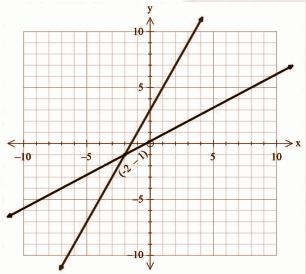(x + y)2 = (-2 – 1)2 = -32 = 9

The value of A = 9.

Question 27: Prove that

[a] [1 + secA] / secA = sin2 A / [1 – cos A]

[b] cos2 θ / [1 – tan θ] + sin3 θ / [sin θ – cos θ] = 1 + sin θ cos θ

OR

Prove that

[a] sin θ / [1 + cos θ] + [1 + cos θ] / sin θ = 2 cosec θ

[b] [sin θ – 2sin3 θ] / [2 cos3 θ – cos θ] = tan θ

Solution:

[a] LHS = (1 + secA) / secA

= (1 + 1 / cosA) / (1 / cosA)

= [(cosA + 1) / cosA] / (1 / cosA)

= cos A + 1

= (1 + cosA) (1 – cosA) / (1 – cosA)

= (1 – cos² A) / (1 – cosA)

= sin² A / (1 – cosA)

= RHS

[b] LHS = cos2 θ / [1 – tan θ] + sin3 θ / [sin θ – cos θ] = 1 + sin θ cos θ

= cos2 θ / [1 – (sin θ / cos θ)] + sin3 θ / [sin θ – cos θ]

= [cos3 θ / cos θ – sin θ] – sin3 θ / [sin θ – cos θ]

= [cos θ – sin θ] [cos2 θ + sin2 θ + sin θ cos θ] / [cos θ – sin θ]

= 1 + sin θ cos θ

= RHS

OR

[a] L.H.S. = sin θ / [1 + cos θ] + [1 + cosθ] / sin θ

= sin2θ + (1 + cos θ)2 / sin θ (1 + cos θ)

= sin2θ + (1 + 2 cos θ + cos2 θ) / sin θ (1 + cos θ)

= (sin2θ + cos2θ) + 1 + 2 cos θ / sin θ (1 + cos θ)

= 1 + 1 + 2 cos θ / sin θ (1 + cos θ)

= 2 + 2 cos θ / sin θ (1 + cos θ)

= 2 (1 + cos θ) / sin θ (1 + cos θ)

= 2 / sin θ

= 2 cosec θ

= RHS

[b] LHS = [sin θ – 2sin3 θ] / [2 cos3 θ – cos θ] = tan θ

(sin θ – 2 sin3 θ) / (2 cos3 θ – cos θ)

= sin θ(1 – 2 sin2 θ) / cos θ (2cos2 θ – 1)

= sin θ [1- 2 (1 – cos2 θ) / cos θ (2cos2 θ – 1)

= sin θ [1 – 2 + 2cos2 θ] / cos θ (2 cos2 θ – 1)

= sin θ (2 cos2 θ – 1) / cos θ (2 cos2 θ – 1)

= sin θ / cos θ

= tan θ

Question 28: If there are four points P (2, –1), Q (3, 4), R (–2, 3) and S (–3, –2) in a plane, then prove that PQRS is not a square but a rhombus.

Solution: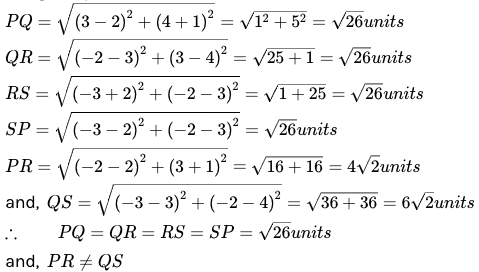This means that PQRS is a quadrilateral whose sides are equal but diagonals are not equal. Thus, PQRS is a rhombus but not a square.

Question 29: Prove that the opposite angles of a cyclic quadrilateral are supplementary or sum is 180º.

OR

Prove that if a chord is drawn from a point of contact of the tangent of the circle then the angle made by this chord with the tangent is equal to the respective alternate angles made by segments with this chord.

Solution: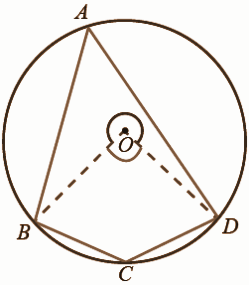O is the centre of the circle.

To prove : ∠BAD + ∠BCD = 180°, ∠ABC + ∠ADC = 180°

Construction: Join OB and OD

Proof:

(i) ∠BAD = (1 / 2) ∠BOD. (The angle subtended by an arc at the centre is double the angle on the circle.)

(ii) ∠BCD = (1 / 2) reflex ∠BOD.

(iii) ∠BAD + ∠BCD = (1/2)∠BOD + (1/2) reflex ∠BOD.

∠BAD + ∠BCD = (1 / 2) (∠BOD + reflex ∠BOD)

∠BAD + ∠BCD = (1 / 2) ⋅ (360°) (Complete angle at the centre is 360°)

(iv) Similarly ∠ABC + ∠ADC = 180°.

OR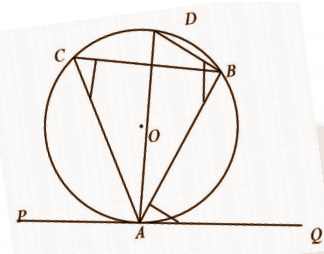∠ACB = ∠ADB —- (i) [circle of segments are equal angles]

∠ABD = 90o —- (ii) [make an angle of semicircle]

∠DAQ = 90o —- (iii) [DA is perpendicular to PQ]

In the triangle ABD,

∠ACB = ∠QAB [from equation (i)]

Question 30: Find the median and mode of the following frequency distribution.

 Class 10 – 25 25 – 40 40 – 55 55 – 70 70 – 85 85 – 100 fi 6 20 44 26 3 1

Solution:

 Class 10 – 25 25 – 40 40 – 55 55 – 70 70 – 85 85 – 100 fi 6 20 44 26 3 1 Cumulative frequency 6 26 70 96 99 100

N = 100

N / 2 = 100 / 2 = 50

The cumulative frequency just greater than 44 is 70 and the corresponding class is 40 – 55.

l = 40, h = 15, f1 = 44, f0 = 20, f2 = 26

Mode = l + (f1 – f0) / (2f1 – f0 – f2) * h

= 40 + {(44 – 20) / (2 * 44 – 20 – 26)} * 15

= 40 + {(24) / (42)} * 15

= 40 + 8.57

= 48.57